# Numbers up to 3-Digits

Go back to  'Number Systems'

In this lesson, explore the world of numbers by going through its various aspects, such as the place value, the significance of zero in a three-digit number, misconceptions. Find answers to questions like what they are, how they are formed, and discover interesting facts around them.

Kids explore the video below. In the video, we can see that Dora and Shelly are in a shop to buy 114 candies. To Dora’s surprise, Shelly counts toffees a hundred times faster than her. Do you know how Shelly did it? Let's watch the video to understand this.

## Lesson Plan

 1 Numbers up to 3-Digits 2 Solved Examples  on Numbers up to 3-Digits 3 Interactive Questions on Numbers up to 3-Digits 4 Free Downloadable Resources on Numbers up to 3-Digits

## Free PDFs for Offline Revision

Get your copy of Numbers up to 3-Digits E-book along with Worksheets and Tips and Tricks PDFs for Free!

## Numbers up to 3-Digits

Let us take one example:

The image is of the sports day, all the kids participated and at last secured their places as first, second, and third.

Start reading the numbers written in the image below from the left you will see 213 which is a three-digit number, that we are going to explore today.In the number 213,

The place value of 3 is ones

The place value of 1 is tens and

The place value of 2 is hundred.

Hey! looking for more such interesting lessons,

Don't forget to go through the table given below, that will land you to your desired lesson.

.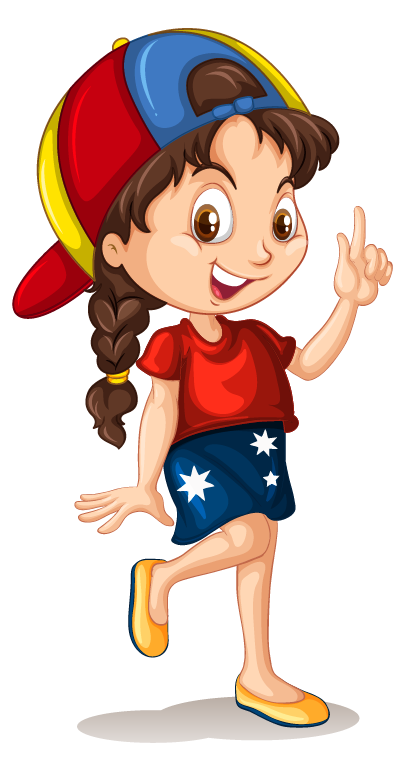Click on any of the short lessons you want to explore!Number up to 2 digits Number up to 4 digits Number up to 5 digits Number up to 6 digits Number up to 7 digits Number up to 8 digits Number up to 9 digits

## Numbers up to 3-Digits

### A Simple Idea: The Place Value of Numbers

Every three-digit number’s value can be found by seeing which place value each digit has.

Let us consider the number 243

The first digit at the rightmost position is said to be in Units place, so they would be multiplied by 1

Hence the product is

\begin{align} ( 3 \times 1 ) = 3 \end{align}

Then the second number is 4, and because it is in Tens place, it is multiplied by 10

The value, therefore, is

\begin{align} ( 4 \times 10 ) = 40 \end{align}

The third number 2 is in the Hundreds Place.

So 2 is multiplied by 100 and its value is

\begin{align} ( 2 \times 100 ) = 200 \end{align}

Therefore,

the number =  \begin{align} 200 + 40 + 3 = 243 \end{align}

Here is an interesting place value game we play at Cuemath!

Form the number with the counters and check your answer if it is correct!

### Decomposing a 3-digit Number

In a three-digit number, there are three place values used – hundred’s, ten’s and units.

Let us take one example to understand it better: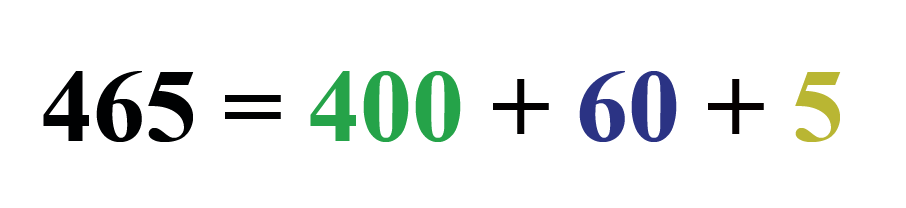Here,

465 is a three-digit number and it is decomposed in the form of a sum of three numbers.

As 5 is at the units place, 60 is at the tens place and 400 is on the hundreds place.

## How is it Important?

### The Significance of Zero in 3-digit numbers

The number zero does not make any contribution to a 3-digit number if it is placed in a position where there are no other non-zero numbers to its left.

So how is 303 different from 033 or even 003?

In 033, the values are

\begin{align} (0 \times 100 ) + ( 3 \times 10 ) + ( 3 \times 1 )\end{align}
\begin{align} = 0 + 30 + 3= 33 \end{align}

which means that the number actually becomes a 2 digit number 33, or in the case of 003, it becomes a single digit number 3

In these two examples, the digits zero do not contribute any value to the number, so the numbers can as well be expressed as 33 or 3.

## Common Mistakes or Misconceptions

Misconception 1: Children make mistakes identifying numbers when there is a zero in the unit’s place or tens place.

Example: When asked to read 130 and 103, students may get confused.
It helps them to model the numbers through Base-10 blocks.
That way they can see the ten’s and one’s place value explicitly.

Misconception 2When asked to write “one hundred twenty-three," students often write 100 first and then attach 23 to it thus ending up with the number “10023”
Fact: This misconception arises due to a superficial understanding of place values.
Using the base-10 blocks or abacus show children that a digit has different values based on its position.

Misconception 3: Sometimes when asked to form the smallest 3-digit number given three digits that include zero, children place the zero in the left-most position.
Fact: This is incorrect.
Zero cannot be in the hundreds place if we are creating a 3-digit number.
e.g. The smallest 3-digit number using all digits of 5, 0, and 7 is 507 and not 057

## Know More about 3 Digit Numbers and its Operation

• Get students to skip count by 10 and 100 to build fluency with 3-digit numbers.
First, start at 100
Then start from any random 3-digit number like 136

• Help children spot the pattern that when skip counting by 10, the digit in the ones place value does not change.
Similarly, when skip counting by 100, the digits in the ones place and tens place does not change

• Use a 100-square grid to build fluency.
Let students spot the pattern that moving one row up or down is the same as skip counting by 10. Moving columns (left or right) increases or decreases numbers by 1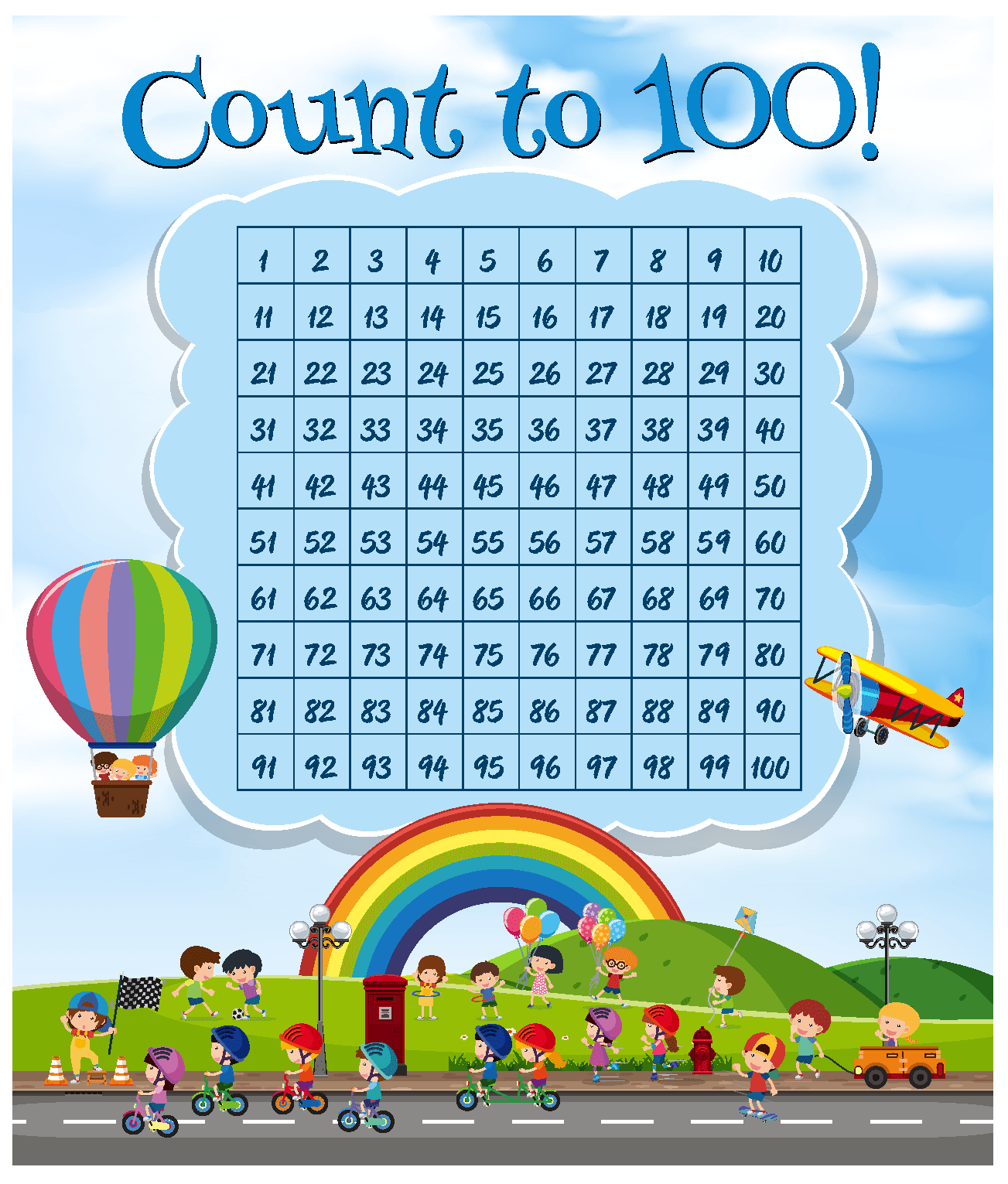• Often children are given three digits and asked to find the largest and smallest number three-digit number using all digits.
The trick here is to arrange all digits in descending order to find the largest number.
To find the smallest number, arrange all digits in ascending order
.
But keep in mind that if zero is one of the digits, it cannot be placed to the left.
E.g. Using the digits 7, 3, and 6, the largest number is 763 (digits in descending order) and the smallest number is 367 (digits in ascending order).
Using the digits 4, 0, and 8, the largest number will be 840 but the smallest 3-digit number is 408 and not 048.

## Solved Examples

 Example 1

Choose the smallest three-digit number from the given options.

a. 101
b. 100
c. 010
d. 111

Solution:

Thus, option c is a 2-digit number.

Now we are left with options a, b and d.

Arranging them in ascending order, we see that 100 is the first 3-digit number.

 $$\therefore$$ Smallest three-digit number is 100
 Example 2

How many 3-digit numbers are there?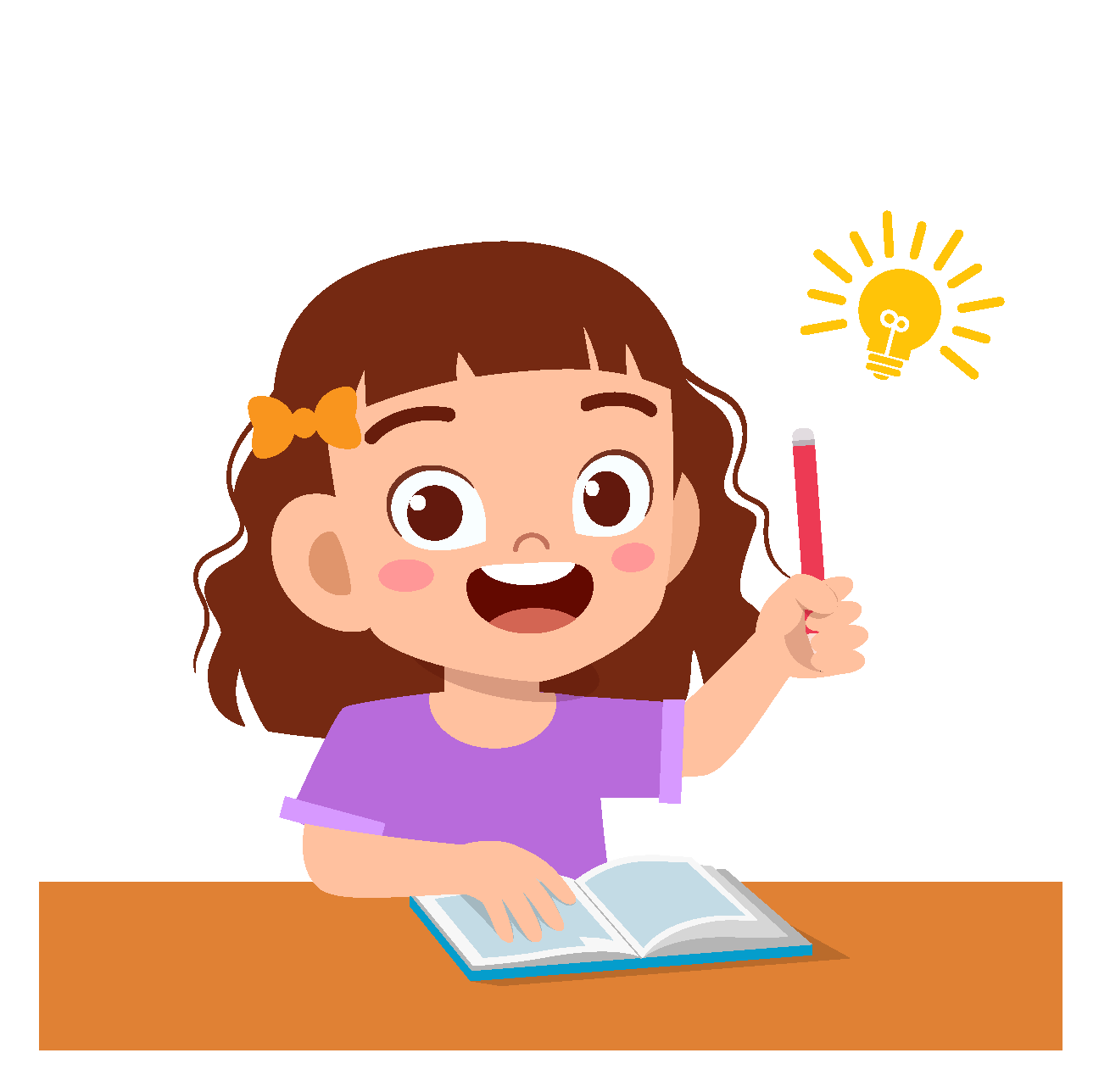Solution:

For a 3-digit number, the hundreds place cannot be 0

It can be any other digit from 1-9

Hence, there can be 9 digits in the hundreds place.

In the tens place and the ones place, there can be any digit from 0-9

Thus, there are $$9 \times 10 \times 10 = 900$$ three digit numbers in all.

 $$\therefore$$ There are 900 three-digit numbers in all.
 Example 3

If the green counters represent hundreds, the blue counters represent tens and the yellow counters represent ones, what is the number represented in the following abacus?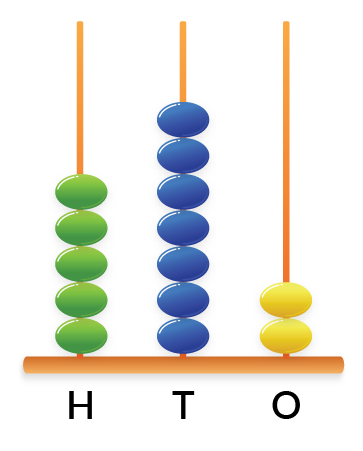Solution:

There are 5 green counters, so there are 5 hundred.

There are 7 blue counters, so there are 7 tens.

There are 2 yellow counters which represent 2 ones.

Therefore, the number is 500 + 70 + 2 = 572

 $$\therefore$$ The abacus represents the number 572
 Example 4

A teacher wrote a puzzle on the board.

"Add the smallest 2 digit number to the smallest 1 digit number. Subtract the sum from one less than the greatest 3 digit number".

Anu said that the answer was 987

Rahul said that it was 997

Who was right?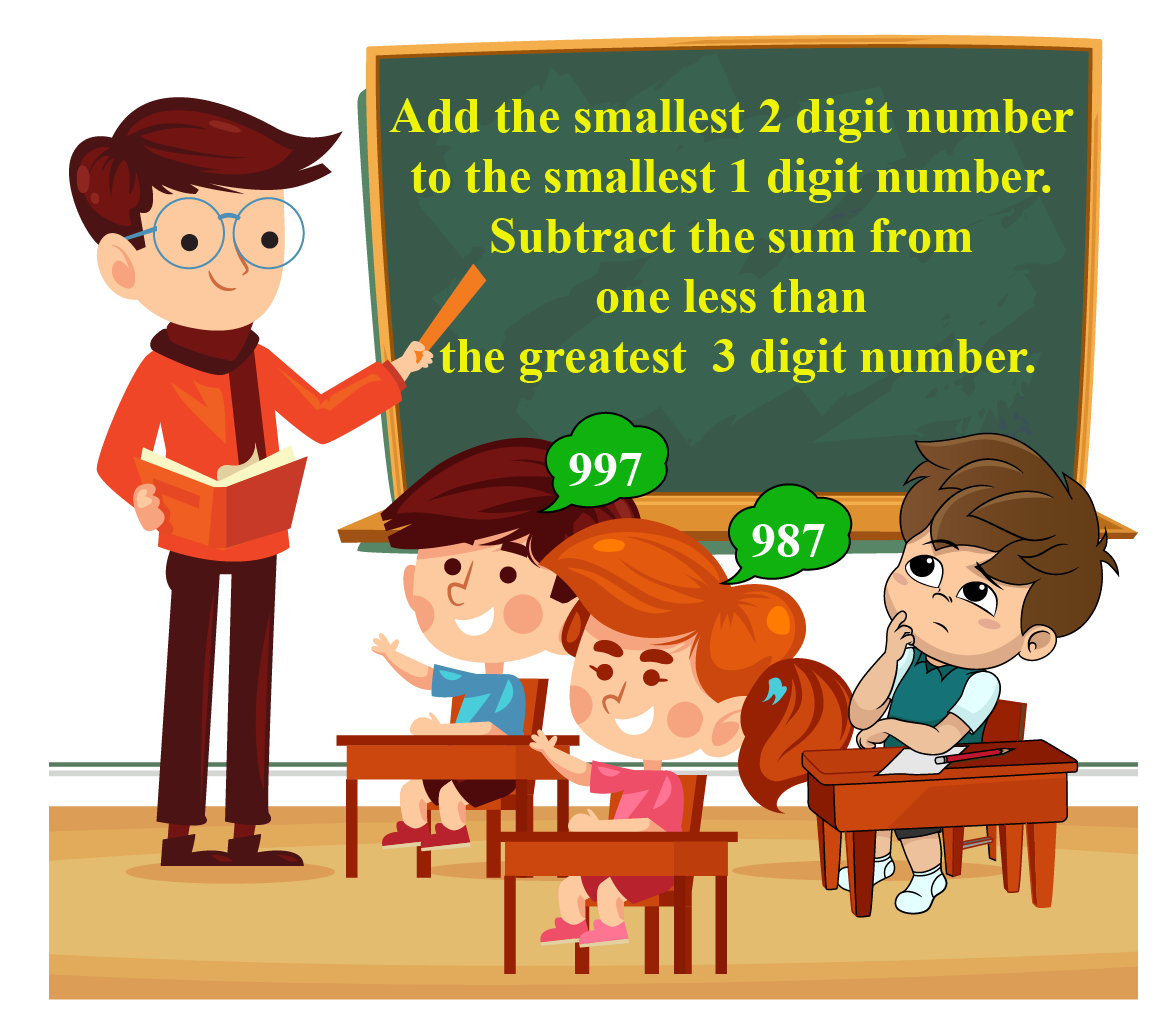Solution:

Smallest 2 digit number = 10

Smallest 1 digit number = 1

Sum of these two numbers = 11

One less than the greatest 3 digit number is 998

Subtracting 11 from 998, we get

$$998 - 11 = 987$$

 $$\therefore$$ Anu's answer is correct.

## Practice Questions

Here are a few activities for you to practice. Select/Type your answer and click the "Check Answer" button to see the result.Think Tank
1.  I am a 3-digit even number.

My ones place is double my tens place and my hundreds place is half my tens place. Who am I?
2. How many 3-digit numbers are even?

## Let's Summarize

So kids hope you loved the lesson and the picture is clear in your mind about the 3 digit numbers, the significance of zero, misconceptions, and you are not left with any doubts if you still have any we would love to hear from you. Drop us your comments in the chat and we would be happy to help.

At Cuemath, our team of math experts is dedicated to making learning fun for our favorite readers, the students! Through an interactive and engaging learning-teaching approach, the teachers explore all angles of a topic. Be it worksheets, online classes, doubt sessions, or any other form of relation, it's the logical thinking and smart approach that we at Cuemath believe in.

## 1. How many 3 digit numbers are there?

There are a total of 900 three-digit numbers.

These include the smallest 3 digit number - 100 to the largest 3 digit number - 999

## 2. Which is the largest 3 digit number?

The largest 3 digit number is 999

Adding 1 more to it will make it a 4 digit number.

## 3. What is the sum of the three largest 3 digit numbers?

The three largest 3 digit numbers are 997, 998, 999

Their sum is 2994

$$997 +998 +999 = 2994$$

3-Digit Numbers Ebook
E-book
3-Digit Numbers -Tips and Tricks
3 digit tips